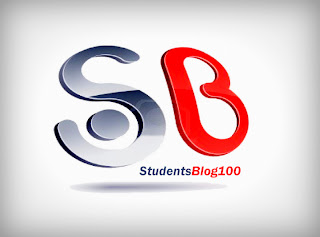CS6702 GRAPH THEORY AND APPLICATIONS SYLLABUS FOR 7TH SEM CSE AND 8TH SEM IT REGULATION 2013 - Anna University Internal marks 2018
ANNA UNIVERSITY CSE/IT SYLLABUS
CS6702 GRAPH THEORY AND APPLICATIONS SYLLABUS
7TH SEMESTER CSE - 8TH SEMESTER IT
REGULATION 2013CS6702 GRAPH THEORY AND APPLICATIONS SYLLABUS
OBJECTIVES:
The student should be made to:
-> Be familiar with the most fundamental Graph Theory topics and results.
-> Be exposed to the techniques of proofs and analysis.

UNIT I INTRODUCTION
Graphs – Introduction – Isomorphism – Sub graphs – Walks, Paths, Circuits –Connectedness –
Components – Euler graphs – Hamiltonian paths and circuits – Trees – Properties of trees – Distance and centers in tree – Rooted and binary trees.

UNIT II TREES, CONNECTIVITY & PLANARITY
Spanning trees – Fundamental circuits – Spanning trees in a weighted graph – cut sets – Properties of cut set – All cut sets – Fundamental circuits and cut sets – Connectivity and separability – Network flows – 1-Isomorphism – 2-Isomorphism – Combinational and geometric graphs – Planer graphs – Different representation of a planer graph.

UNIT III MATRICES, COLOURING AND DIRECTED GRAPH
Chromatic number – Chromatic partitioning – Chromatic polynomial – Matching – Covering – Four color problem – Directed graphs – Types of directed graphs – Digraphs and binary relations – Directed paths and connectedness – Euler graphs.

UNIT IV PERMUTATIONS & COMBINATIONS
Fundamental principles of counting - Permutations and combinations - Binomial theorem - combinations with repetition - Combinatorial numbers - Principle of inclusion and exclusion - Derangements - Arrangements with forbidden positions.

UNIT V GENERATING FUNCTIONS
Generating functions - Partitions of integers - Exponential generating function – Summation operator - Recurrence relations - First order and second order – Non-homogeneous recurrence relations - Method of generating functions.

TOTAL: 45 PERIODS

OUTCOMES:

Upon Completion of the course, the students should be able to:
-> Write precise and accurate mathematical definitions of objects in graph theory.
-> Use mathematical definitions to identify and construct examples and to distinguish examples from non-examples.-> Validate and critically assess a mathematical proof.-> Use a combination of theoretical knowledge and independent mathematical thinking in creative-> investigation of questions in graph theory.-> Reason from definitions to construct mathematical proofs.

TEXT BOOKS:
1. Narsingh Deo, “Graph Theory: With Application to Engineering and Computer Science”, Prentice Hall of India, 2003.
2. Grimaldi R.P. “Discrete and Combinatorial Mathematics: An Applied Introduction”, Addison Wesley, 1994.

REFERENCES:
1. Clark J. and Holton D.A, “A First Look at Graph Theory”, Allied Publishers, 1995.
2. Mott J.L., Kandel A. and Baker T.P. “Discrete Mathematics for Computer Scientists and Mathematicians” , Prentice Hall of India, 1996.
3. Liu C.L., “Elements of Discrete Mathematics”, Mc Graw Hill, 1985.
4. Rosen K.H., “Discrete Mathematics and Its Applications”, Mc Graw Hill, 2007.